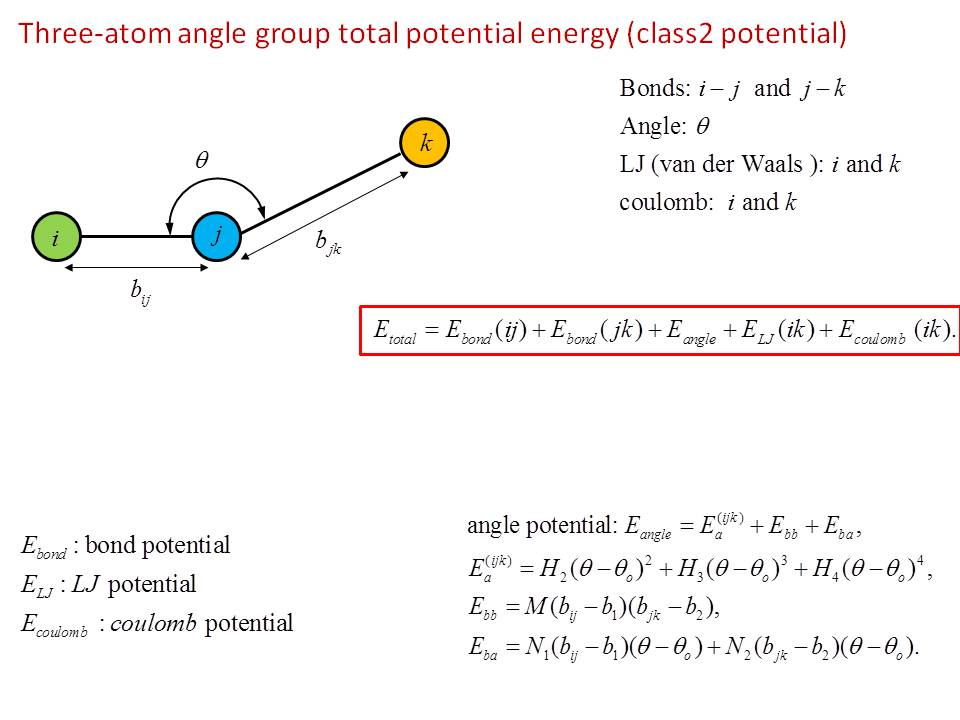# Question about total potential energy forms (class2 potential)

Dear Everyone,

I have a question on describing the total potential energy for class2 force field.

Let me take a 3-atom angle group as an example.

Please see the figure attached for total energy form.

I am not sure if Etotal is the one used in Lammps. If not, can anyone please correct me?

Thank you for your time,

Lili ZhangThe potential energy for the angle term
in class2 is given in a formula on the
doc page: doc/angle_class2.html

Steve

Hello Everyone,

Thanks Steve for your comment.

I am confused about the “total potential energy” and the “angle energy term in the doc page” for a 3- atom angle group for Class2 force field.

The total potential energy = angle energy term (in the doc page) + all bond energies + LJ energy+…

Or the total potential energy = the angle energy term (in the doc page) only?

Thank you for your help,

Lili Zhang

The formula on the angle class2 doc page is only the energy
for the angle term in the total potential energy.

Steve